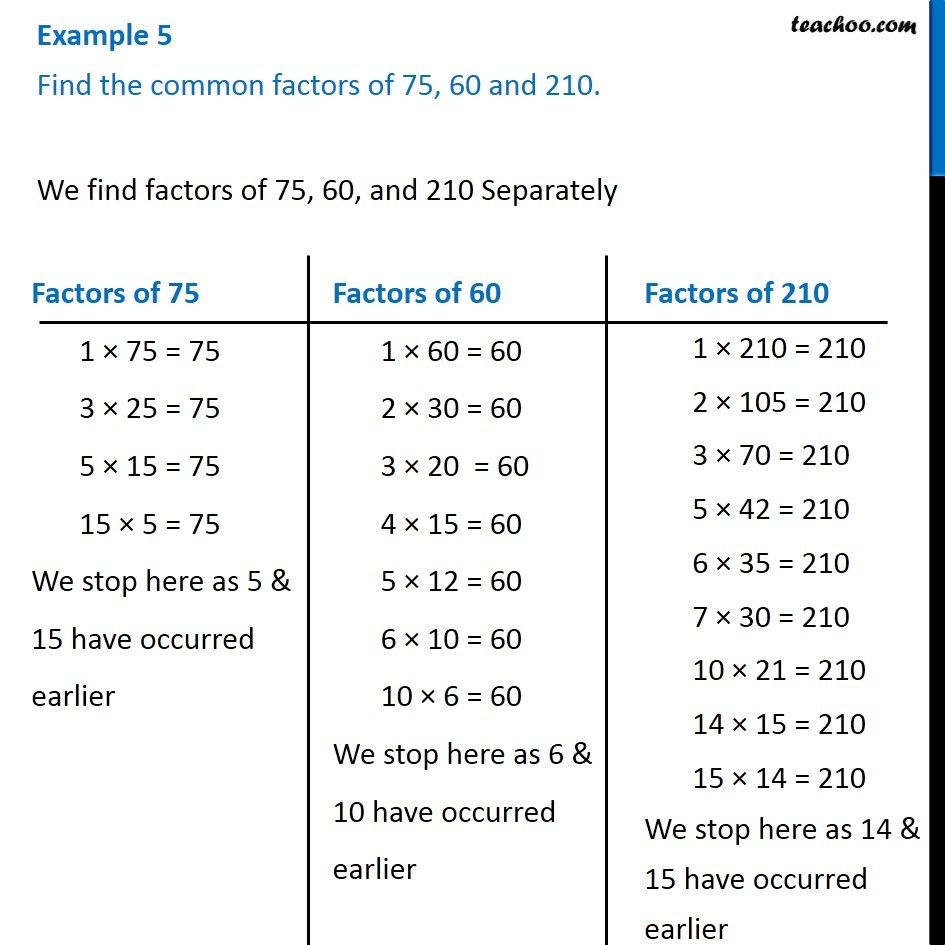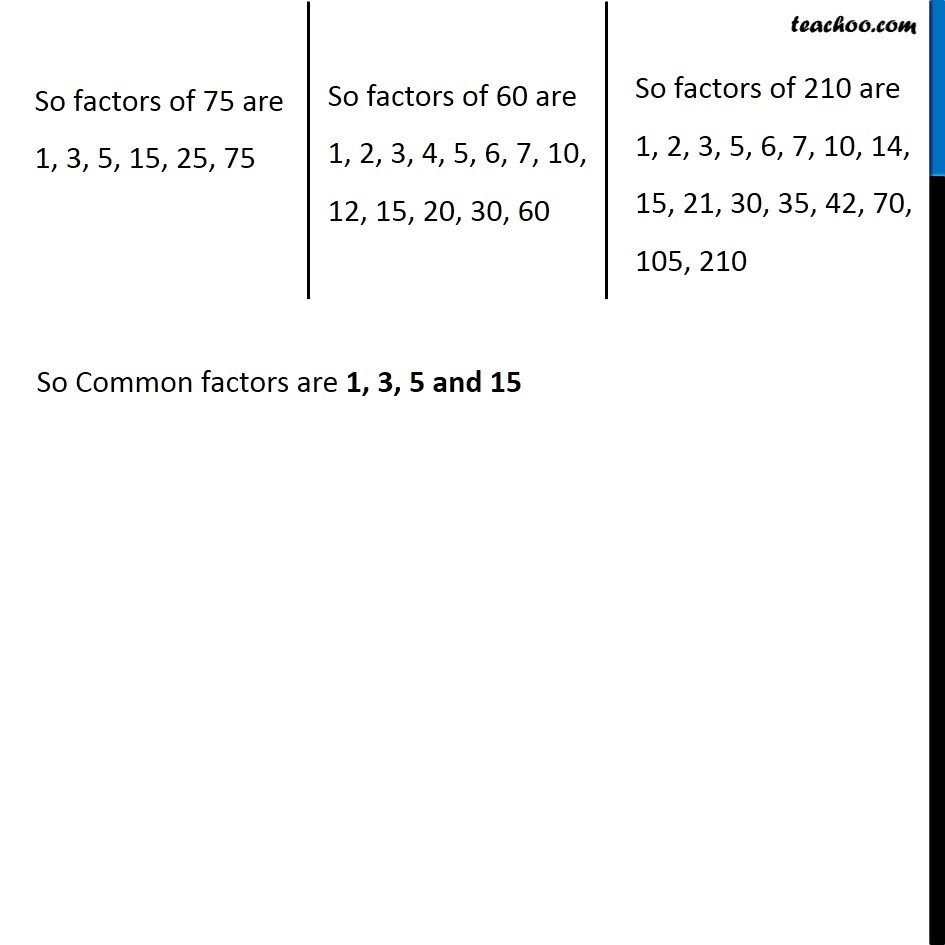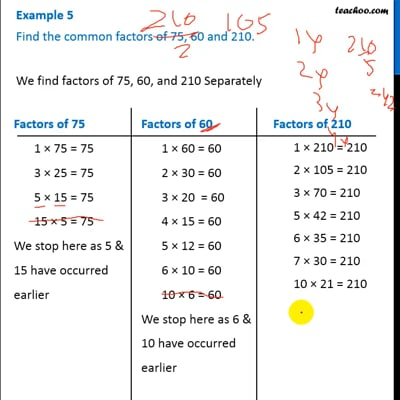Examples

Chapter 3 Class 6 Playing with Numbers
Serial order wiseThis video is only available for Teachoo black users

Get live Maths 1-on-1 Classs - Class 6 to 12

### Transcript

Example 5 Find the common factors of 75, 60 and 210.We find factors of 75, 60, and 210 Separately Factors of 75 1 × 75 = 75 3 × 25 = 75 5 × 15 = 75 15 × 5 = 75 We stop here as 5 & 15 have occurred earlier Factors of 60 1 × 60 = 60 2 × 30 = 60 3 × 20 = 60 4 × 15 = 60 5 × 12 = 60 6 × 10 = 60 10 × 6 = 60 We stop here as 6 & 10 have occurred earlier Factors of 210 1 × 210 = 210 2 × 105 = 210 3 × 70 = 210 5 × 42 = 210 6 × 35 = 210 7 × 30 = 210 10 × 21 = 210 14 × 15 = 210 15 × 14 = 210 We stop here as 14 & 15 have occurred earlier So factors of 75 are 1, 3, 5, 15, 25, 75 So factors of 60 are 1, 2, 3, 4, 5, 6, 7, 10, 12, 15, 20, 30, 60 So factors of 210 are 1, 2, 3, 5, 6, 7, 10, 14, 15, 21, 30, 35, 42, 70, 105, 210 So Common factors are 1, 3, 5 and 15